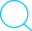4 May 2018

# Avoiding pit-falls in PyTorch- Never create a torch.tensor from an existing container of tensors (previously called Variables)

#### Never create a new `torch.tensor` /`variable` from an existing list/ tuple/ sequence/ container of `tensors`/`variables`

Unless you don’t mind loosing the history of computations done on the `tensors`/`variables` in the list/tuple/sequence/container. In other words, unless you do not need the computation graph to include the operations done on those `tensors`/`variables` in the list/tuple/sequence/container, do not create a new `torch.tensor` from the list/tuple/sequence/container hoping to preserve the operations and use the automatic differentiation.

In some other, simpler words, if you want to use the automatic differentiation goodness of PyTorch to compute gradients with respect to some `tensors`/`variables` that are part of the chain of computations you do with the output(s) of a neural network with learn-able parameters, and if for convenience you have those `tensors`/`variables` packed into an easy to use python container (like a list, tuple ,`collections.named_tuple` etc.), do not convert the python container to a torch tensor or variable by using `torch.tensor(CONTAINER_NAME)`. This will destroy the computation histories associated with the `tensors`/`variables` inside the container. Even if you use `torch.tensor(CONTAINER_NAME, requires_grad=True)`, the history of computations will be lost!

Let’s look at some example with code to understand that better.

For an example, let’s say you want to train an Actor-Critic policy gradient based reinforcement learning agent where you are using a neural network implemented in PyTorch to approximate the Critic. As you may know, the Critic can be trained using the Temporal Difference (TD) targets computed using the n-step or 1-step returns. You would usually calculate the error with this simple equation: `td_error = td_target - critic_predicted_value` or `td_error = ((td_target - critic_predicted_value)^2).mean()` if you want to use MSE. For calculating the n-step TD error, you may use the previously computed (cached) value predictions by the critic network along with other information (like the observation, action, log probability of action etc) which you may have stored as a list/tuple/namedtuple or some other python container for ease of use. Now when you want to calculate the `td_error`, since tuple or list do not support arithmetic subtraction, you might be tempted to convert the `critic_predicted_value` into a torch tensor/variable like shown below:

``````# TD targets computed and returned as a tuple
td_targets = compute_n_step_returns(rewards, next_state, done, gamma)
# Critic's predictoins returned as a tuple from a previusly computed namedtuple
critic_predicted_values = n_step_trajectory.value_s
# Can't do this:
td_error = td_targets - critic_predicted_values
# Can do this:
td_error = torch.tensor(td_target) - torch.tensor(critic_predicted_values)
# But, the above will destroy the history of computations done to obtain the
# critic_predicted_value and so it's grad_fn will be None and no gradients will be computed during the backward pass

# The following line will also have the same problem as above:
td_error = torch.tensor(td_target) - torch.tensor(critic_predicted_values, requires_grad=True)

# The correct way is to do something like this:
td_error = [target - prediction for target, prediction in zip(td_targets, critic_predicted_values)]
# And then you can take the mean:
td_error = np.mean(td_error)  # This returns a torch tensor and preserves the computations

``````

Another example when the loss methods in PyTorch’s `torch.nn.functional` module is used to calculate the loss. We’ll use the `mse_loss`in this example but it applies to any other loss calculation operation as you can guess:

``````# TD targets computed and returned as a tuple
td_targets = compute_n_step_returns(rewards, next_state, done, gamma)
# Critic's predictoins returned as a tuple from a previusly computed namedtuple
critic_predicted_values = n_step_trajectory.value_s
# Can't do this:
td_error = torch.nn.functional.mse_loss(critic_predicted_values, td_targets)
# Can't do this because the first argument should need a gradient:
td_error = torch.nn.functional.mse_loss(torch.tensor(critic_predicted_values), td_targets)
# Can do this:
# But, the above will destroy the history of computations done to obtain the
# critic_predicted_value and so it's grad_fn will be None and no gradients will be computed during the backward pass

# The correct way is to do something like this:
td_error = [torch.nn.functional.mse_loss(prediction, target) for prediction, target in zip(critic_predicted_values, td_targets)]
# And then you can take the mean:
td_error = torch.stack(td_error).mean()  # This returns a torch tensor and preserves the computations
``````

Hope that helps someone when they are trying to figure out why their neural network implementation in PyTorch is :

• not learning or improving or
• not receiving any parameter updates or
• not propagating the gradients as expected